# Schneider method

(diff) ← Older revision | Latest revision (diff) | Newer revision → (diff)

The name "Schneider method" arises from the solution, by Th. Schneider [a5] in 1934, to Hilbert's seventh problem (cf. also Hilbert problems): Ifis a non-zero algebraic number,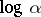a non-zero logarithm ofandan irrational algebraic number, then the number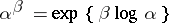is transcendental (cf. also Transcendental number).

One main idea in Schneider's proof is to investigate values of a function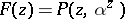at points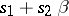(), whereis a polynomial with algebraic coefficients. Assuming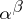is algebraic, a non-zero polynomialis constructed so thatvanishes at many such points; this construction is based on Dirichlet's box principle or pigeon hole principle (the Thue–Siegel lemma, cf. also Transcendency, measure of; Dirichlet principle). A clever computation of a determinant is an essential tool of Schneider's proof.

A slight modification of the same argument yields the so-called six exponentials theorem [a2], [a4]: If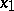,are two complex numbers that are linearly independent over(cf. also Linear independence) and if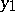,,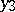are three complex numbers that are linearly independent over, then at least one of the six numbers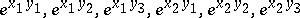is transcendental.

In relation with this statement, one of the simplest open (as of 2000) problems which would follow from the conjecture that "linearly independent logarithms of algebraic numbers are algebraically independent" is the four exponentials conjecture: If,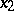are two complex numbers that are linearly independent overand if,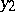are also two complex numbers that are linearly independent over, then at least one of the four numbers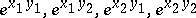is transcendental.

Both S. Lang [a2] and K. Ramachandra [a4] have given general statements concerning the simultaneous algebraic values of analytic functions by means of Schneider's method. Typically, these statements are efficient for functions satisfying functional equations, for instance when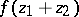,andare algebraically dependent. This method yields transcendence results as well as results of algebraic independence on values of exponential, elliptic or Abelian functions; more generally it applies to the arithmetic study of commutative algebraic groups.

Schneider's method can be extended to several variables, and then yields partial results related to the Leopoldt conjecture on the-adic rank of the units of an algebraic number field [a6]. It also gives lower bounds for the ranks of matrices whose entries are linear combinations with algebraic coefficientsof logarithms of algebraic numbers [a3], a special case of which is Roy's strong six exponentials theorem: Ifis a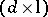-matrix, with, whose entries are linear combinations of logarithms of algebraic numbers, with rows linearly independent over the field of algebraic numbers and with columns linearly independent over the field of algebraic numbers, then the rank ofis at least.

An extension of Schneider's method also provides sharp measures for linear independence of logarithms of algebraic numbers [a1].

How to Cite This Entry:
Schneider method. Encyclopedia of Mathematics. URL: http://encyclopediaofmath.org/index.php?title=Schneider_method&oldid=11823
This article was adapted from an original article by Michel Waldschmidt (originator), which appeared in Encyclopedia of Mathematics - ISBN 1402006098. See original article